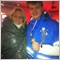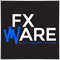# Profits per currency pair indicator1284

Hi all!

I'm trying to display in an indicator the current profit level and the % of equity that it represents.

Actually, I'm testing it across 17 pairs and it this moment there are open trades on 14 of them, so I would expect to have information displayed for all of those.

However, for some reason, I can only get 10 pairs displayed and I don't know why.

Any suggestion? This is my code!

```   string CommentString="";

double freeMargin=AccountInfoDouble(ACCOUNT_EQUITY);

string symbols[],symb;
double profit[];
bool existe=false;
for(int i=OrdersTotal()-1;i>=0;i--)
{
{
symb=OrderSymbol();

for(int j=0;j<ArraySize(symbols);j++)
{
if(symb==symbols[j])
{
existe=true;
}
}
if(!existe)
{
ArrayResize(symbols,ArraySize(symbols)+1);
ArrayResize(profit,ArraySize(profit)+1);
symbols[ArraySize(symbols)-1]=symb;

for(int k=OrdersTotal()-1;k>=0;k--)
{
{
if(OrderSymbol()==symbols[ArraySize(symbols)-1])
{
profit[ArraySize(profit)-1]+=NormalizeDouble(OrderProfit(),2);
}
}
}
}
}
}
for(int i=0;i<ArraySize(symbols);i++)
{
CommentString+="Profits on "+symbols[i]+": "+NormalizeDouble(profit[i],2)+" ("+MathFloor(MathAbs(NormalizeDouble(profit[i],2)/freeMargin*10000))/100+"%) "+"\n";
}1284

Just realized that the "existe" variable should go inside the second loop and this was the issue.

I'll leave the correction here, in case anyone needs it in the future.

```        for(int j=0;j<ArraySize(symbols);j++)
{
existe = false;
if(symb==symbols[j])
{
existe=true;
}
}```1284

I'm having another problem and its related to the information displayed.

With the calculation of the profit and the % it represents, sometimes it shows "0.07000000000000000000000001%" (a huge decimal). Is there a way to truncate it?

I tried with MathFloor and NormalizeDouble in many variations and so far no success.1579

Pedro Severin:

I'm having another problem and its related to the information displayed.

With the calculation of the profit and the % it represents, sometimes it shows "0.07000000000000000000000001%" (a huge decimal). Is there a way to truncate it?

I tried with MathFloor and NormalizeDouble in many variations and so far no success.

Use StringToDouble to represent it with a fixed number of digits.9

lippmaje:
Use StringToDouble to represent it with a fixed number of digits.458

Pedro Severin:

I'm having another problem and its related to the information displayed.

With the calculation of the profit and the % it represents, sometimes it shows "0.07000000000000000000000001%" (a huge decimal). Is there a way to truncate it?

I tried with MathFloor and NormalizeDouble in many variations and so far no success.

StringFormat would be suitable to prepare such a string - in the beginning formatting rules may seem a bit difficult but learning them is worth the time. Rules are best described in PrintFormat function documentation.1284

Thank you all!

At last I used DoubleToString. I don't know why, but StringToDouble didn't work :(.

I will leave the code here, if anyone needs it or want to makes any improvements :)

This will display all the symbols and sum all the profits that you have across all symbols by symbol, displaying the % of equity that represents. At the bottom, it also displays an overview of the account and the current drawdown as a %.

Positive numbers are displayed in lime and negative in red.

I hope you like this! This is the first "indicator" I make :D

```//+------------------------------------------------------------------+
//|                                                     Drawdown.mq4 |
//|                                     Pedro Pablo Severin Honorato |
//|                                             https://www.mql5.com |
//+------------------------------------------------------------------+
#property copyright "Pedro Pablo Severin Honorato"
#property version   "1.00"
#property strict
#property indicator_chart_window

string profits_on="Profits on: ";
string balance="balance";
string drawdown="drawdown";
string patrimonio="patrimonio";
string accprof="profits";
//+------------------------------------------------------------------+
//| Custom indicator initialization function                         |
//+------------------------------------------------------------------+
int OnInit()
{
//--- indicator buffers mapping

//---
return(INIT_SUCCEEDED);
}
//+------------------------------------------------------------------+
//|                                                                  |
//+------------------------------------------------------------------+
void OnDeinit(const int reason)
{
Comment("");
ObjectDelete(profits_on);
ObjectDelete("=");
ObjectDelete(balance);
ObjectDelete(patrimonio);
ObjectDelete(drawdown);
ObjectDelete(accprof);
for(int i=0;i<30;i++)
{
ObjectDelete(i);
}
}
//+------------------------------------------------------------------+
//| Custom indicator iteration function                              |
//+------------------------------------------------------------------+
int OnCalculate(const int rates_total,
const int prev_calculated,
const datetime &time[],
const double &open[],
const double &high[],
const double &low[],
const double &close[],
const long &tick_volume[],
const long &volume[],
{
//---

ObjectDelete(profits_on);
ObjectCreate(profits_on,OBJ_LABEL,0,0,0,0);
ObjectSet(profits_on,OBJPROP_CORNER,1);
ObjectSet(profits_on,OBJPROP_XDISTANCE,100);
ObjectSet(profits_on,OBJPROP_YDISTANCE,15);

ObjectSetText(profits_on,"Profits on: ",10,"Arial",White);

string DepositCurrency=AccountInfoString(ACCOUNT_CURRENCY);

double freeMargin=AccountInfoDouble(ACCOUNT_EQUITY);

string symbols[],symb;
double profit[];
bool existe;
for(int i=OrdersTotal()-1;i>=0;i--)
{
{
symb=OrderSymbol();

for(int j=0;j<ArraySize(symbols);j++)
{
existe=false;
if(symb==symbols[j])
{
existe=true;
break;
}
}
if(!existe)
{
ArrayResize(symbols,ArraySize(symbols)+1);
ArrayResize(profit,ArraySize(profit)+1);
symbols[ArraySize(symbols)-1]=symb;

for(int k=OrdersTotal()-1;k>=0;k--)
{
{
if(OrderSymbol()==symbols[ArraySize(symbols)-1])
{
profit[ArraySize(profit)-1]+=NormalizeDouble(OrderProfit(),2);
}
}
}
}
}
}

for(int i=0;i<19;i++)
{
ObjectDelete(i);
}

for(int i=0;i<ArraySize(symbols);i++)
{
ObjectCreate(i,OBJ_LABEL,0,0,0,0);
ObjectSet(i,OBJPROP_CORNER,1);
ObjectSet(i,OBJPROP_XDISTANCE,100);
ObjectSet(i,OBJPROP_YDISTANCE,15+20*(i+1));
color col=(profit[i]>0)?Lime:
(profit[i]<0)?Red:White;

ObjectSetText(i,symbols[i]+": "+ DoubleToString(NormalizeDouble(profit[i],2),2)+" ("+DoubleToString(NormalizeDouble(MathFloor(MathAbs(NormalizeDouble(profit[i],2)/freeMargin*10000))/100,2),2)+"%)",10,"Arial",col);

if(NormalizeDouble(MathFloor(MathAbs(NormalizeDouble(profit[i],2)/freeMargin*10000))/100,2)>5)
{
}
}
ObjectDelete("=");
ObjectDelete(balance);
ObjectDelete(patrimonio);
ObjectDelete(drawdown);
ObjectDelete(accprof);

ObjectCreate("=",OBJ_LABEL,0,0,0,0);
ObjectSet("=",OBJPROP_CORNER,1);
ObjectSet("=",OBJPROP_XDISTANCE,100);
ObjectSet("=",OBJPROP_YDISTANCE,15+20*(ArraySize(symbols)+1));
ObjectSetText("=","====================",10,"Arial",White);

ObjectCreate(balance,OBJ_LABEL,0,0,0,0);
ObjectSet(balance,OBJPROP_CORNER,1);
ObjectSet(balance,OBJPROP_XDISTANCE,100);
ObjectSet(balance,OBJPROP_YDISTANCE,15+20*(ArraySize(symbols)+2));
ObjectSetText(balance,"Account Balance: "+AccountBalance(),10,"Arial",White);

ObjectCreate(patrimonio,OBJ_LABEL,0,0,0,0);
ObjectSet(patrimonio,OBJPROP_CORNER,1);
ObjectSet(patrimonio,OBJPROP_XDISTANCE,100);
ObjectSet(patrimonio,OBJPROP_YDISTANCE,15+20*(ArraySize(symbols)+3));
ObjectSetText(patrimonio,"Account Equity: "+AccountEquity(),10,"Arial",White);

ObjectCreate(accprof,OBJ_LABEL,0,0,0,0);
ObjectSet(accprof,OBJPROP_CORNER,1);
ObjectSet(accprof,OBJPROP_XDISTANCE,100);
ObjectSet(accprof,OBJPROP_YDISTANCE,15+20*(ArraySize(symbols)+4));
ObjectSetText(accprof,"Account Profits: "+DoubleToString(NormalizeDouble(AccountProfit(),2),2),10,"Arial",White);

ObjectCreate(drawdown,OBJ_LABEL,0,0,0,0);
ObjectSet(drawdown,OBJPROP_CORNER,1);
ObjectSet(drawdown,OBJPROP_XDISTANCE,100);
ObjectSet(drawdown,OBJPROP_YDISTANCE,15+20*(ArraySize(symbols)+5));
ObjectSetText(drawdown,"Account Drawdown: "+DoubleToString(NormalizeDouble(AccountProfit()/AccountEquity()*100,2),2)+"%",10,"Arial",White);

//--- return value of prev_calculated for next call
return(rates_total);
}
//+------------------------------------------------------------------+
```1579

Pedro Severin:

Thank you all!

At last I used DoubleToString. I don't know why, but StringToDouble didn't work :(.

Of course, I meant DoubleToString but mistyped it.